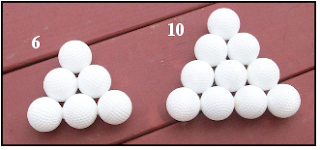## Friday, July 10, 2009

### Figurate Numbers-Part 2-Pyramid Numbers

I introduced figurate numbers (see the May 2009 folder) as a hands-on way to introduce kids to the mathematical beauty and number patterns that can be discovered by arranging objects according to a rule.

As learned in the previous post, the rule for forming triangular numbers is to successively sum the counting (natural) numbers, starting with the number 1. How the first four triangular numbers are formed is shown in the following diagram.In one of the April 2009 posts I described Paula’s Puzzle. Four golf balls are glued to form a triangular-based pyramid. Five of these form a larger, 20-ball pyramid (see below). The puzzle is to figure out how to build the larger pyramid from the five smaller pyramids.

Is there a simple relationship between the triangular numbers and Paula’s Puzzle? The answer is “yes” and the kids can easily demonstrate the relationship.

Materials

You need a glue gun and twenty plastic practice golf balls. The golf balls cost a couple of buck a dozen and are available at any of the chain stores.

If your kids constructed Paula’s Puzzle, then they already know how to safely use a hot glue gun. If not, this is a good time for them to learn.

Have them build the second triangular number (3) by gluing together two golf balls and then gluing the third in place as shown in this picture.

Help them to build the golf ball models for the triangular numbers 6 and 10.Once the kids have built the models, have them arrange the models in order, as shown below.
Ask them to build successive triangular based pyramids. It will be so easy for them to do this that they will think it’s trivial. Oh, if only we could convince them much of math was as easy to do!

Summary

Triangular numbers are made by successively summing the set of counting numbers and pyramid numbers (tetrahedral numbers) are made by successively summing triangular numbers.

Give the kids a blank sheet of paper and help them make a record of the relationship between the triangular numbers and the pyramid numbers. Use the following table as a guide.

Here’s a short video that illustrates how pyramid numbers are built from triangular numbers.

1.2.